# XY Plotting

Home » Knowledge Base » LS-Prepost » Other » XY Plotting

•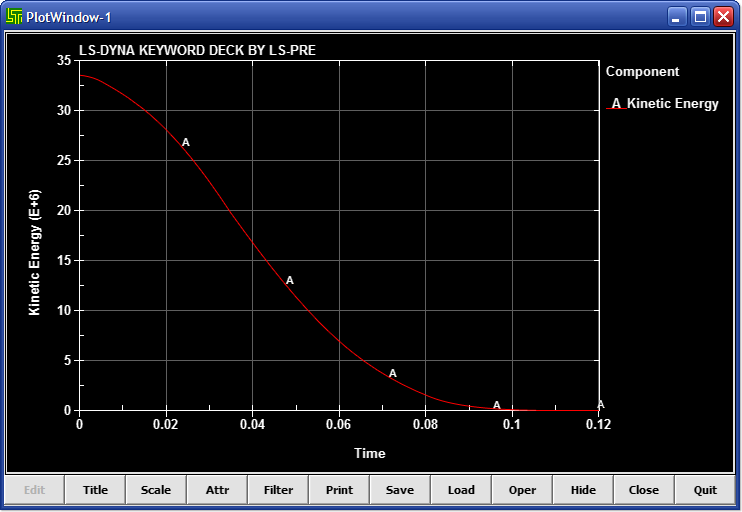• Figure 1 – XYPlot Interface

• Left and Right Menus (Common)
•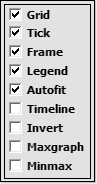•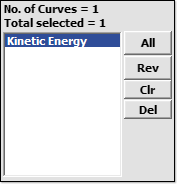• Figure 2 – Left Menu

Select all curves in the list

Reverse curve selection

Clear curve selection

Remove selected curve from list

•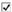Grid – Add/remove grid lines in XY-Plot window
•Tick – Add/remove tick marks in XY-Plot window
•Frame – Add/remove frame in XY-Plot window
•Legend – Add/remove legend in XY-Plot window
•Autofit – Toggle Automatically Fit data in XY-Plot window (on/off)
•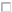Timeline – Toggle time line in XY-Plot window
•Invert – Invert background color in XY-Plot window
•Maxgraph – Use all of XY-Plot window to display data
•MinMax – Add/remove min/max label in XY-Plot window

This function has been deprecated.

Set and change titles/axis labels

•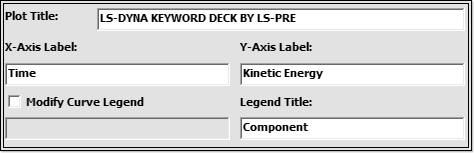• Figure 4 – XYPlot Title
• Plot Title: Input plot title

• X-Axis label: Input x-axis label
• Y-Axis label: Input y-axis label

•Modify Curve Legend – Modify name in legend

• Legend Title: Input legend title

Set and change offset/scale of curves

•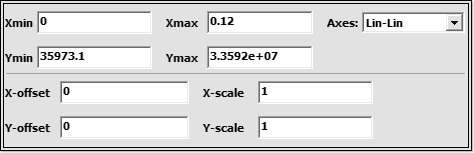• Figure 5 – XYPlot Scale
• Xmin: Input min value for x-axis
• Xmax: Input max value for x-axis
• Ymin: Input min value for y-axis
• Ymax: Input max value for y-axis
• Axes: Select logarithmic/linear scale

• X-offset: Input offset value for X-axis
• Y-offset: Input offset value for Y-axis
• X-scale: X axis scale factor
• Y-scale: Y axis scale factor

Change attributes of XY-Plot

•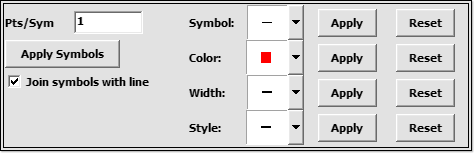• Figure 6 – XYPlot Attr
• Pts/Sym: Input # of points per symbol
•Join symbols with line – Toggle lines between symbols

• Symbol: Select symbol style
• Color: Select curve and symbol color
• Width: Select line width
• Style: Select line style

Apply individual changes

Reset individual changes

Set curve filtering

•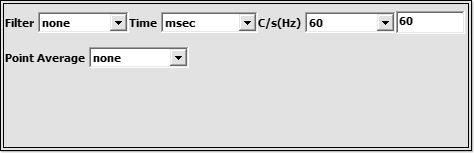• Figure 7 – XYPlot Filter
• Filter: Select filter to apply

• Time: Select time units

• C/s(Hz): Select/Enter frequency

• Point Average: Select number of points to average
• (example, PA=3: avg of ordinate values taken every
• 3 points, and all 3 points are given the avg value)

Open print dialog

Write XY-Plot data to a file

•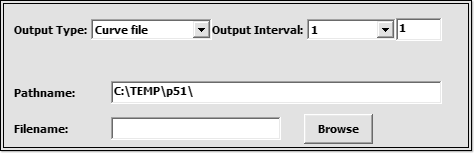• Figure 8 – XYPlot Save
• Output Type: Select data output type
• Output Interval: Select/Enter # of time steps between outputs

• Pathname: Enter path to file save location
• Filename: Enter filename

Browse to select file save location

Write XY-Plot data to given filename

Load XY-Plot data from a file

•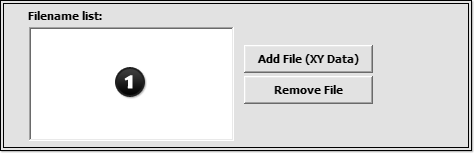• Figure 9 – XYPlot Load
• Filename list: Select file to show stored data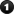Add a file to the filename list

Remove a file from the filename list

Open selected data files

Process XY-Plot data

•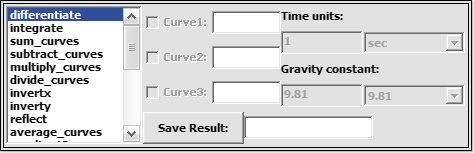• Figure 10 – XYPlot Oper
• Operations List: Select operation to apply

•Curve 1 – Pick/Enter first curve for operation
•Curve 2 – Pick/Enter second curve for operation
•Curve 3 – Pick/Enter third curve for operation

• Time Units: Select/Enter HIC time units
• Gravity Constants: Select/Enter HIC gravity constants

Save resultant curve as XYPlot data file

• Note: Fast Fourier Transforms will be performed only on data visible in the plot window, so zooming in will effect the results. (this applies to the “fft” and “fft_radix” operations)

Hide buttons (click in window and hit Esc to restore)

Close the plot window

Close and delete plot window from the memory